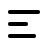51md.cn

51秒懂电商创业网

www.51md.cn

# 三、实验步骤

1.准备图片

2.导入模块

```import time
from  tkinter import  filedialogimport tkinter as t
from PIL import Image
from tkinter import messageboximport os```

3.制作软件界面

`self.tl=[] #存放图片的列表self.aa='' #存放音频的地址self.fg=os.path.join(os.path.expanduser("~"), 'Desktop') #桌面地址self.root=t.Tk()self.root.title('淘宝主图视频生成器v1.0')#主界面标题self.root.iconbitmap('1.ico')  #添加主界面标题图片self.root.geometry('700x600') #主界面大小self.root.attributes('-alpha',0.9) #主界面透明度self.root.wm_attributes('-topmost',1) #主界面置顶self.tt=t.Text(self.root,width=75,height=30) #文本框#主界面中的所有按钮和一些标签部件self.b1=t.Button(self.root,text='打开图片文件',font =("宋体",13,'bold'),command=self.open_file)self.b3=t.Button(self.root,text='打开音频文件',font =("宋体",13,'bold'),command=self.adio)self.b7=t.Button(self.root,text='打开文件并批量修改',font =("宋体",13,'bold'),command=self.xg)self.b8=t.Button(self.root,text='查看照片',font =("宋体",13,'bold'),command=self.ck)self.l1=t.Label(self.root,text='视频文件名：')self.e=t.Entry(self.root)self.b2=t.Button(self.root,text='生成主图视频',font =("宋体",13,'bold'),command=self.video)self.b4=t.Button(self.root,text='播放音频文件',font =("宋体",13,'bold'),command=self.play)self.b5=t.Button(self.root,text='清空文本框内容',font =("宋体",13,'bold'),command=self.close)self.b6=t.Button(self.root,text='预览视频内容',font =("宋体",13,'bold'),command=self.player)self.l2=t.Label(self.root,text='视频分辨率：')self.l3=t.Label(self.root,text='长：')self.e1=t.Entry(self.root,width=5)self.l4=t.Label(self.root,text='宽：')self.e2=t.Entry(self.root,width=5)self.tt.place(x=10,y=50)self.b1.place(x=100,y=10)self.l1.place(x=120,y=460)self.e.place(x=200,y=460)self.b2.place(x=200,y=550)self.b3.place(x=240,y=10)self.b4.place(x=550,y=120)self.b5.place(x=550,y=200)self.b6.place(x=550,y=300)self.l2.place(x=120,y=500)self.l3.place(x=200,y=500)self.l4.place(x=280,y=500)self.e1.place(x=230,y=500)self.e2.place(x=310,y=500)self.b7.place(x=380,y=10)self.b8.place(x=580,y=10)self.root.mainloop()`4.软件功能具体实现过程

1）打开图片文件，打开音频文件

```#选择用于主图视频的图片aa=filedialog.askopenfilenames(title='选择所有主图文件',filetypes=[('png','PNG'),('jpg','JPG'),('All Files', '*')])#将它的后缀提取出来为下一步做准备aa=self.tl

2）打印视频生成时输出的日志到文本框

ffmpeg生成视频时会有一串日志，但是这个日志是无法用popen捕获的，所以我们得用dos 捕获将它重定向到某个文件，然后我们在读取文件中的内容到文本框，所以我们需要这么做：

```if os.path.exists('1.txt'): #先判断是不是有这个文件
try:
f=open(os.path.join(self.fg,'1.txt'),'r',encoding='utf-8') #打开文件
except:
messagebox.showinfo(title='提示',message='-----成功生成主图视频文件失败----') #提示
finally:
self.tt.insert('insert','\n')
messagebox.showinfo(title='提示',message='-----成功生成主图视频文件成功----\n 文件名为：%s.mp4'%self.e.get())
f.close() #关闭文件流else:
af=open(os.path.join(self.fg,'1.txt'),'w') #没有这个文件就创建
af.close() #关闭文件流```

3）运用ffmpeg 生成视频

`os.popen('ffmpeg -r 0.3 -t 300 -i {}/%d.png -i {} -strict -2 -f mp4 -s {}x{} {} >{} 2>&1'.format(ab,self.aa,str(self.e1.get()),str(self.e2.get()),os.path.join(self.fg,self.e.get()+'.mp4'),os.path.join(self.fg,'1.txt')))`

`ffmpeg这个都知道，程序名-r:视频帧-t 视频结束事件-i 后面一般接 图片或者音频 视频名称-strict 2表示 为了使用aac音频编码而设置的标准-f  mp4 视频输出格式-s 视频分辨率`

5、预览视频

`os.popen(self.e.get()+'.mp4')`

# 四、项目总结### 相关阅读

#### 拼多多店铺流量不多怎么办（解析店铺流量少的原因）

2022-10-23 16:55:08

#### 分享用精准标签流量(提升店铺宝贝排名安全上首页)

2022-08-22 18:17:42

#### 拼多多出评改销量最新方法都有哪些(解读注意事项什么)

2022-08-22 18:14:42

#### 淘宝安全补单平台是去哪里找的？正规补单平台推荐

2022-08-22 18:11:26